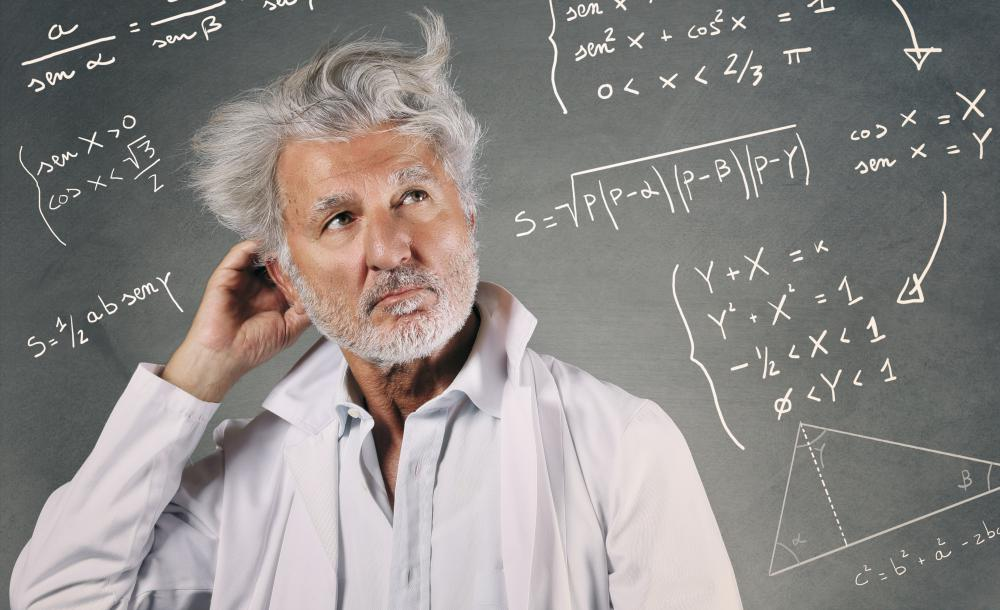What are Binary Numbers?

D.M. Abrecht
D.M. Abrecht

The word "binary" refers to a system made up of two parts, like a binary star. Binary numbers are not different than the numbers you're used to; they're just represented differently — with 1s and 0s only. While binary numbers are used in a number of fields, they are most commonly used is electrical and computer applications.

The most common system for representing numbers is not the binary system; it's the decimal system. Also known as base-10, the decimal system which uses ten digits — 0 through 9. Each place within a number corresponds to a power of 10. Thus, the decimal number 546.23 is equal to: (5 x 102) + (4 x 101) + (6 x 100) + (2 x 10-1) + (3 x 10-2)In a general sense, binary systems can be anything which offers only two options, not necessarily limited to numerical systems.

There are many other systems of numeric notation, however; the binary system, also known as base-2, is one. Binary numbers use only the digits 0 and 1. Each place in the number corresponds to a power of 2. Therefore, the binary number 11100, for example, would be represented in the following decimal format: (1 x 24) + (1 x 23) + (1 x 22) + (0 x 21) + (0 x 20) = 16 + 8 + 4 + 0 + 0 = 28

Clearly, the decimal system is a more compact system of notation than the binary system. Still, the binary system has some unique properties that make it quite useful for certain operations, including those used by digital computers. Since each binary digitbit for short — has only two possible states, it is easily represented with an electrical switch with two positions. The number "1" represents the switch being on, or "yes," while the number "0" represents the switch being off, or "no."

Binary arithmetic can be performed using a small number of simple rules, making it possible to calculate numbers using only a handful of electrical gates. For instance, to multiply two digits together, you only need to remember the following:

0 x 0 = 0
0 x 1 = 0
1 x 0 = 0
1 x 1 = 1

The two-value system for representing binary numbers can also be seen to correspond to the two truth values used in symbolic logic. Consider the following truth tables utilizing the logical operator "AND:"

F AND F = F
F AND T = F
T AND F = F
T AND T = T

If you replace "F" with "0" and "T" with "1," it becomes clear that the logical operator "AND" is equivalent to the multiplication sign in binary arithmetic. The other mathematical operations can likewise be exchanged for logical operations. Since logical operators are easy to represent in computer circuitry, it becomes possible to build an electrical device that can perform arithmetic. Doing math this way is known as "Boolean algebra" after its discoverer, 19th century mathematician George Boole.

In computer memory, a block of eight bits is called a byte. A byte can represent the numbers 00000000 through 11111111, which is 0 through 255 in the decimal system. Different computing architectures can handle different numbers of bits in a single computation; such a group of bits is called a word. A word is often a multiple of eight bits, with 16-, 32-, and 64-bit words being the most common.

You might also Likeanon133203

So then in the binary numeral system, 0 is represented as 0 and 1 is represented as 1. That's where the simplicity stops. 3 in the binary system is represented as: 11, 4 is represented as 100, and 5 is represented as 101. It's really a whole new way of looking at numbers!

What I would like to know is, when you are taking a college class and you received a book for that class, why doesn't it explain binary and decimal as it is explained in this article. What a difference simplicity makes!

buddy

bjurszeni1

What I would like to know is, when you are taking a college class and you received a book for that class, why doesn't it explain binary and decimal as it is explained in this article. What a difference simplicity makes!

averagejoe

So then in the binary numeral system, 0 is represented as 0 and 1 is represented as 1. That's where the simplicity stops. 3 in the binary system is represented as: 11, 4 is represented as 100, and 5 is represented as 101. It's really a whole new way of looking at numbers!